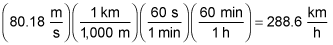##### Physics I: Practice Problems For DummiesIn physics, you can relate an object's mass, velocity, and kinetic energy. For example, if two birds of different masses are flying at the same speed, you can calculate how much more kinetic energy the heavier one uses than the lighter one.

Here are some practice questions that you can try.

## Practice questions

1. Two birds, a sparrow and a cardinal, fly east with the same speed. If the sparrow's kinetic energy is denoted K, what is the cardinal's kinetic energy in terms of K if it is four times as massive as the sparrow?

2. How fast is a 28-gram bullet moving if it has a kinetic energy of 90 joules?

Give your answer in kilometers per hour, rounded to two significant digits.

The following are the answers to the practice questions:

1. 4K

An object's kinetic energy is directly proportional to its mass. If the mass is multiplied by a factor of 4, then so is the kinetic energy.

2. 290 km/h

First convert the mass into "correct" physics units: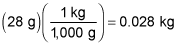Then use the formula for kinetic energy,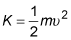where m is the mass of the object and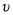is its velocity.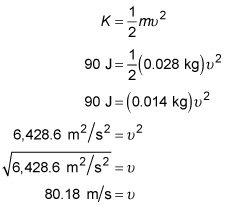Finally, convert into the correct units: